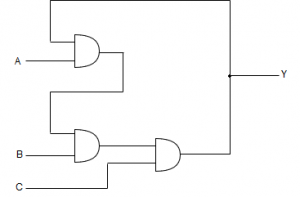# GATE | GATE CS 1999 | Question 33

Consider the circuit shown below . In a certain steady state, the line Y is at ‘ 1 ‘. What are the possible values of A, B and C in this state?a). A=0, B=0, C=1

b). A=0, B=1, C=1

c).  A=1, B=0, C=1

d). A=1, B=1, C=1
(A) b only
(B) Both a and b
(C) Both a and c
(D) a, c and d

Explanation:

Quiz of this Question

My Personal Notes arrow_drop_up
Article Tags :

Be the First to upvote.

Please write to us at contribute@geeksforgeeks.org to report any issue with the above content.